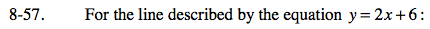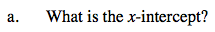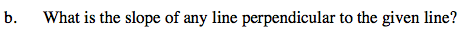### Home > CAAC > Chapter 8 > Lesson 8.2.2 > Problem8-57

8-57.The x-intercept is where y = 0.

Subtract 6 from both sides.

Divide by 2 on both sides.

y = 2x + 6
0 = 2x + 6

−6 = 2x

−3 = x

The x-intercept is (−3, 0).The slope of a line perpendicular to another line is the negative reciprocal of the other line's slope.

Example:
Line (a): y = 4x + 7
m = 4

$\text{The line perpendicular to line (a) has the slope }-\frac{1}{4}.$